## Octav Olteanu: Invariant Subspaces and Invariant Balls of Bounded Linear Operators, p. 261-271

### Abstract:

Let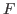be a complex Banach space,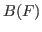the algebra of all bounded operators acting on,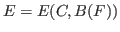the Fréchet space of all entire functions from the complex plane into, endowed with the topology of uniform convergence on the compact subsets in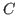. Let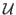be a subset ofsuch that the product of any two elements fromis still an element of. For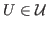, one denotes by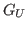the linear continuous operator from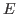intodefined by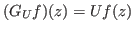,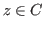,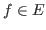. One proves that there exist common invariant subspaces of all operators in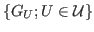. In particular, there exist common invariant subspaces for all operators in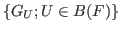. One describes the elements of a dense subspace of such an invariant subspace. On the other side, a differential equation with operator - valued solution is discussed, in the Hilbert space setting. A related example is given, where an invariant subspace appears as a kernel of a differential operator. This subspace stands for a hyperinvariant subspace related to an arbitrary operator in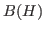. Finally, the invariance of the unit ball in some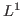spaces in terms of polynomials is discussed. To this end, polynomial approximation on unbounded subsets is applied.

Key Words: invariant subspace problem, invariant balls, bounded operators, sandwich type theorems for operators, approximation

2010 Mathematics Subject Classification: Primary 47A15,
Secondary 41A10, 47A63